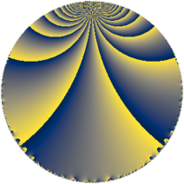# Properties

 Label 108.4.iLevel $108$ Weight $4$ Character orbit 108.i Rep. character $\chi_{108}(13,\cdot)$ Character field $\Q(\zeta_{9})$ Dimension $54$ Newform subspaces $1$ Sturm bound $72$ Trace bound $0$

# Related objects

## Defining parameters

 Level: $$N$$ $$=$$ $$108 = 2^{2} \cdot 3^{3}$$ Weight: $$k$$ $$=$$ $$4$$ Character orbit: $$[\chi]$$ $$=$$ 108.i (of order $$9$$ and degree $$6$$) Character conductor: $$\operatorname{cond}(\chi)$$ $$=$$ $$27$$ Character field: $$\Q(\zeta_{9})$$ Newform subspaces: $$1$$ Sturm bound: $$72$$ Trace bound: $$0$$

## Dimensions

The following table gives the dimensions of various subspaces of $$M_{4}(108, [\chi])$$.

Total New Old
Modular forms 342 54 288
Cusp forms 306 54 252
Eisenstein series 36 0 36

## Trace form

 $$54q + 12q^{5} - 48q^{9} + O(q^{10})$$ $$54q + 12q^{5} - 48q^{9} - 87q^{11} + 234q^{15} + 204q^{17} - 12q^{21} + 96q^{23} - 216q^{25} + 27q^{27} + 318q^{29} - 54q^{31} + 63q^{33} + 6q^{35} + 66q^{39} + 867q^{41} - 513q^{43} - 306q^{45} - 1548q^{47} + 594q^{49} - 1368q^{51} - 1068q^{53} - 1269q^{57} - 1218q^{59} - 54q^{61} + 30q^{63} + 96q^{65} - 2997q^{67} + 1476q^{69} - 120q^{71} - 216q^{73} + 732q^{75} + 3480q^{77} + 2808q^{79} + 3348q^{81} + 4464q^{83} + 2160q^{85} + 4824q^{87} + 4029q^{89} + 270q^{91} + 1164q^{93} - 1650q^{95} - 3483q^{97} - 5076q^{99} + O(q^{100})$$

## Decomposition of $$S_{4}^{\mathrm{new}}(108, [\chi])$$ into newform subspaces

Label Dim. $$A$$ Field CM Traces $q$-expansion
$$a_2$$ $$a_3$$ $$a_5$$ $$a_7$$
108.4.i.a $$54$$ $$6.372$$ None $$0$$ $$0$$ $$12$$ $$0$$

## Decomposition of $$S_{4}^{\mathrm{old}}(108, [\chi])$$ into lower level spaces

$$S_{4}^{\mathrm{old}}(108, [\chi]) \cong$$ $$S_{4}^{\mathrm{new}}(27, [\chi])$$$$^{\oplus 3}$$$$\oplus$$$$S_{4}^{\mathrm{new}}(54, [\chi])$$$$^{\oplus 2}$$